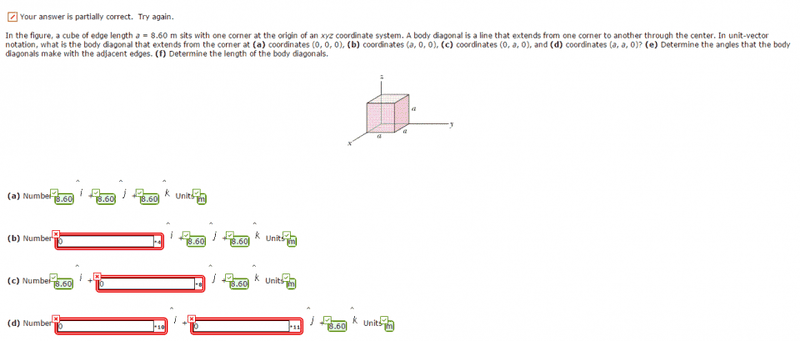# Body diagonals -- unit vector notation

Iron_Man_123
Member warned to complete the template and show details of the effort made

## Homework Statement

I'm fully convinced that the zero values make sense, yet they are wrong, can somebody please explain why is that the caseN/A

## The Attempt at a Solution

Attempt in the image above

Homework Helper
Gold Member
2022 Award
(a,0,0) is the bottom, left and front vertex in the diagram, right? Where is the other end of the diagonal from there?

Iron_Man_123
(a,0,0) is the bottom, left and front vertex in the diagram, right? Where is the other end of the diagonal from there?

It's at the top right corner of the image, which has 0 x-coordinate, am i mistaken?

Homework Helper
Gold Member
2022 Award
It's at the top right corner of the image, which has 0 x-coordinate, am i mistaken?
That's right. The vector along that diagonal is the difference between the endpoints, as vectors.

Iron_Man_123
That's right. The vector along that diagonal is the difference between the endpoints, as vectors.

However, I wrote zero in the i component in the answer space to part (b), does that mean the computer system is wrong and I am right?